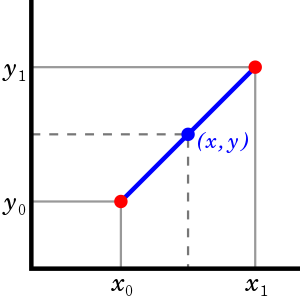# Interpolation

### Linear Interpolation​

In mathematics, linear interpolation is a method of curve fitting using linear polynomials to construct new data points within the range of a discrete set of known data points.### Polynomial Interpolation​

In numerical analysis, polynomial interpolation is the interpolation of a given data set by the polynomial of lowest possible degree that passes through the points of the dataset.

Lagrange Interpolation

If we are given enough values at specific points, we can use Lagrange interpolation to find underlying polynomial

Spline Interpolation

Spline interpolation is a form of interpolation where the interpolant is a special type of piecewise polynomial called a spline. That is, instead of fitting a single, high-degree polynomial to all of the values at once, spline interpolation fits low-degree polynomials to small subsets of the values, for example, fitting nine cubic polynomials between each of the pairs of ten points.

## Bezier Interpolation​

Signature Smoothing using bezier curve interpolation.

## Bonus​

Finding if signature are similar using Frechet distance

Intutive Understanding of Frechet distance